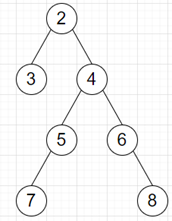# Program to find second deepest node in a binary tree in python

Suppose we have a binary tree; we have to find the depth of the second deepest leaf. If there are multiple deepest leaves, the second deepest leaf node will be the next highest one. As we know the root has a depth of 0.

So, if the input is likethen the output will be 1, as the second deepest node is 3.

To solve this, we will follow these steps:

• if root is null, then
• return null
• nodes := a new list
• insert root at the end of nodes
• count := 0, prev := 0, now := 0
• while nodes is not empty, do
• new := a new list
• flag := True
• for each node in nodes, do
• if flag is true and (left of node is null) and (right of node is null), then
• prev := now
• now := count
• flag := False
• if left of node is not null, then
• insert left of node at the end of new
• if right of node is not null, then
• insert right of node at the end of new
• nodes := new
• count := count + 1
• return prev

Let us see the following implementation to get better understanding:

## Example

Live Demo

class TreeNode:
def __init__(self, data, left = None, right = None):
self.data = data
self.left = left
self.right = right

class Solution:
def solve(self, root):
if root is None:
return None
nodes = []
nodes.append(root)
count = 0
prev = 0
now = 0
while nodes:
new = []
flag = True
for node in nodes:
if flag and (not node.left) and (not node.right):
prev = now
now = count
flag = False
if node.left:
new.append(node.left)
if node.right:
new.append(node.right)
nodes = new
count += 1
return prev

ob = Solution()
root = TreeNode(2)
root.left = TreeNode(3)
root.right = TreeNode(4)
root.right.left = TreeNode(5)
root.right.right = TreeNode(6)
root.right.left.left = TreeNode(7)
root.right.right.right = TreeNode(8)
print(ob.solve(root))

## Input

root = TreeNode(2)
root.left = TreeNode(3)
root.right = TreeNode(4)root.right.left = TreeNode(5)root.right.right = TreeNode(6)root.right.left.left = TreeNode(7)
root.right.right.right = TreeNode(8)

## Output

1

Updated on: 26-Nov-2020

212 Views